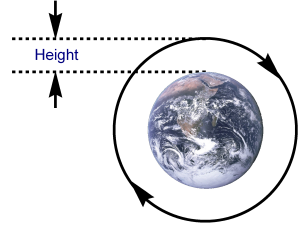# Earth Orbit Calculator

Created by Luis Hoyos
Last updated: Jun 25, 2022

Use our earth orbit calculator to find the velocity or period of an earth satellite, given its height above sea level:

• Calculating the orbital speed of a satellite is crucial to keep it orbiting around the earth and avoid it flying away from earth, or even worse, crashing against it. The resulting momentum of this speed, combined with gravity , makes possible this orbital movement.
• Additionally, calculating the orbital period of an earth's satellite is necessary to know its position at any moment.

## Formula to calculate the orbital speed of a satellite

With the following formula, you can calculate the velocity of a satellite orbiting the earth in a circular orbit:

$\small \text{orbital speed} = \sqrt{\frac{G \cdot M_\text{E}}{(R_\text{E}+h)}}$

where:

• $G$ – Earth's gravitational constant;
• $M_\text{E}$ – Earth's mass;
• $R_\text{E}$ – Earth's radius; and
• $h$ – Perpendicular distance of the satellite from the surface of the earth.

The previous formula is used by this earth orbit calculator when choosing the "Speed of the satellite" option. If you enter the height of the object spinning the earth, the calculator will account for the earth's mass, radius, and gravitational constant and will give you the satellite velocity.

## Formula to calculate the orbital period of an earth's satellite

To calculate the orbital period of an earth's satellite, use this formula:

$\small \text{orbital period} = 2\pi \sqrt{\frac{(R_\text{E}+h)^3}{G \cdot M_\text{E}}}$

The previous one is also the formula used by this calculator. In the earth orbit calculator, select the "Period of satellite rotation" option and input the object's height. You'll have the answer without worrying about the earth's mass, radius, and gravitational constant.

## Example of how to use this earth orbit calculator

Let's calculate the orbital speed of the International Space Station (ISS):

• We know the ISS orbits at 400 km above the earth's surface.
• Select the "Speed of the satellite" option and input 400 km in the "Height" box. The obtained orbital speed should be 7.672 km/s.

Do you wonder how fast an object would fall from high heights? Check the terminal velocity formula to see how air resistance can limit the final speed!

Luis Hoyos
Select
Period of satellite rotation
Height
mi
Orbital period
hrsHeight of the satellite from the Earth.
People also viewed…

### Rocket thrust

Learn how to calculate the thrust of a rocket with our handy tool.